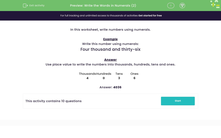# Write the Words in Numerals (2)

In this worksheet, students write number using numerals from the written words.Key stage:  KS 2

Curriculum topic:   Number: Number and Place Value

Curriculum subtopic:   Read and Write Numerals/Words to 1000

Popular topics:   Place Value worksheets

Difficulty level:#### Worksheet Overview

In this activity, we will be learning to write numbers using numerals.

We can use place value to write numbers accurately in numerals.

Let's take the number six hundred and twenty-one as an example.

We know that the number has 6 hundreds, 2 tens and a one.

 Hundreds Tens Ones 6 2 1

We can write this using place value: 600 + 20 + 1

So, the number in numerals is 621.

Let's try an example question together.

Example

Write this number using numerals:

Four hundred and thirty-six.

We can use place value to write the numbers into hundreds, tens and ones.

 Hundreds Tens Ones 4 3 6

400 + 30 + 6

So, the number four hundred and thirty-six can be written in numerals as 436.

### What is EdPlace?

We're your National Curriculum aligned online education content provider helping each child succeed in English, maths and science from year 1 to GCSE. With an EdPlace account you’ll be able to track and measure progress, helping each child achieve their best. We build confidence and attainment by personalising each child’s learning at a level that suits them.

Get started